# COMPSCI 102 Introduction to Discrete Mathematics Cantors Legacy

• Slides: 55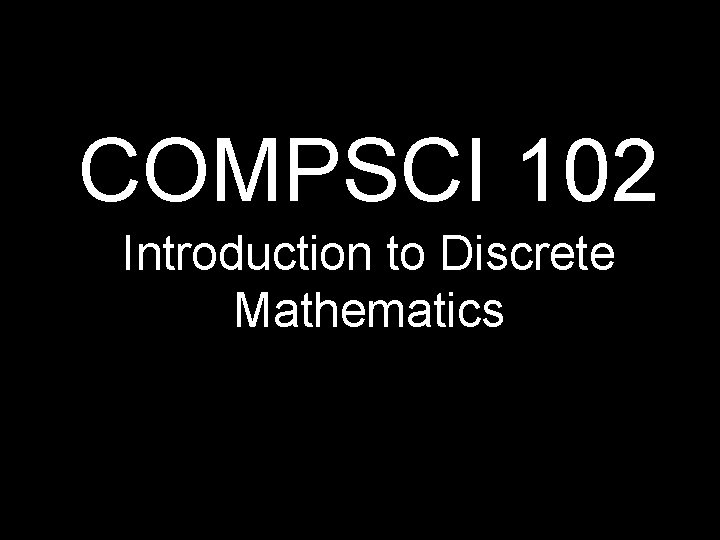COMPSCI 102 Introduction to Discrete Mathematics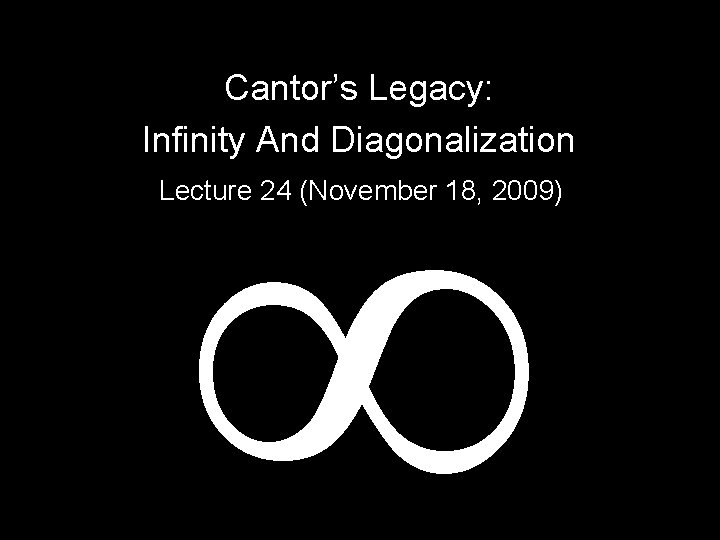Cantor’s Legacy: Infinity And Diagonalization Lecture 24 (November 18, 2009)The Theoretical Computer: no bound on amount of memory no bound on amount of time Ideal Computer is defined as a computer with infinite RAM You can run a Java program and never have any overflow, or out of memory errors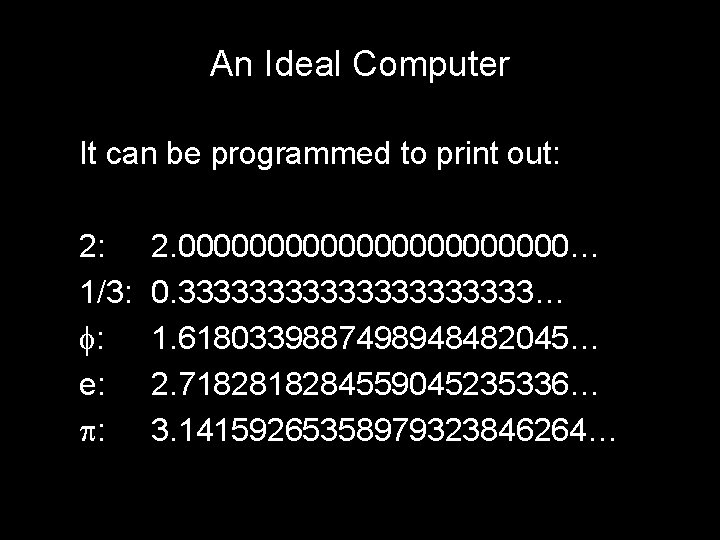An Ideal Computer It can be programmed to print out: 2: 1/3: : e: : 2. 00000000000… 0. 3333333333… 1. 6180339887498948482045… 2. 718284559045235336… 3. 14159265358979323846264…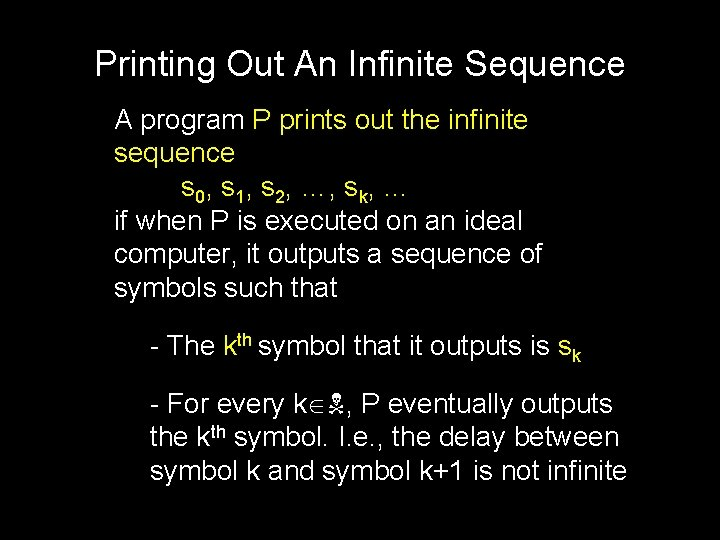Printing Out An Infinite Sequence A program P prints out the infinite sequence s 0, s 1, s 2, …, sk, … if when P is executed on an ideal computer, it outputs a sequence of symbols such that - The kth symbol that it outputs is sk - For every k , P eventually outputs the kth symbol. I. e. , the delay between symbol k and symbol k+1 is not infiniteComputable Real Numbers A real number R is computable if there is a (finite) program that prints out the decimal representation of R from left to right. Thus, each digit of R will eventually be output. Are all real numbers computable?Describable Numbers A real number R is describable if it can be denoted unambiguously by a finite piece of English text 2: “Two. ” : “The area of a circle of radius one. ” Are all real numbers describable?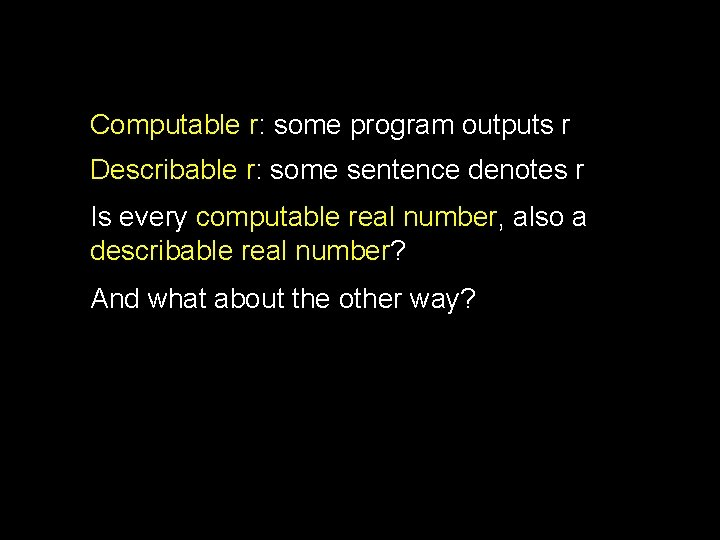Computable r: some program outputs r Describable r: some sentence denotes r Is every computable real number, also a describable real number? And what about the other way?Computable Describable Theorem: Every computable real is also describable Proof: Let R be a computable real that is output by a program P. The following is an unambiguous description of R: “The real number output by the following program: ” P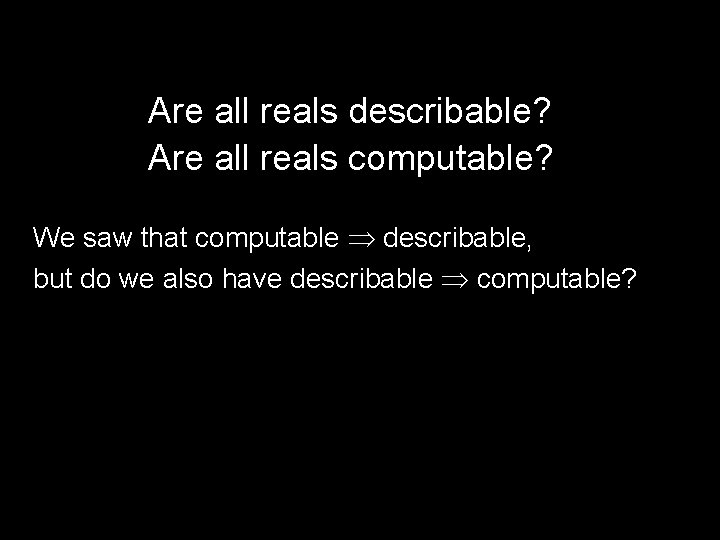Are all reals describable? Are all reals computable? We saw that computable describable, but do we also have describable computable?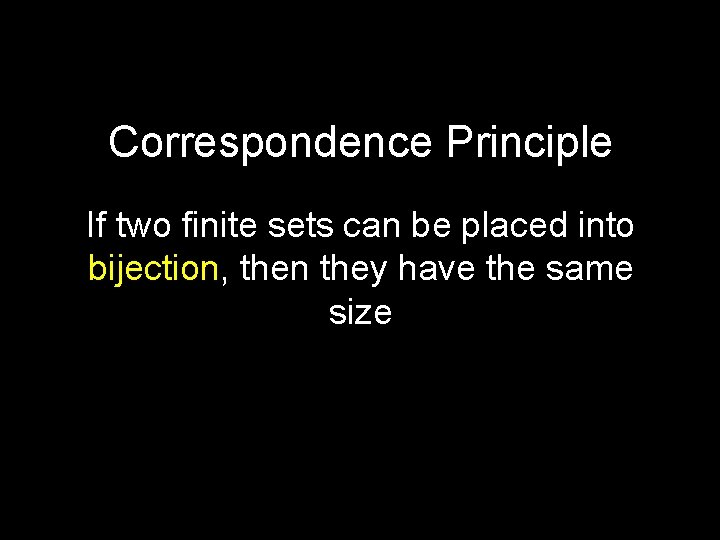Correspondence Principle If two finite sets can be placed into bijection, then they have the same size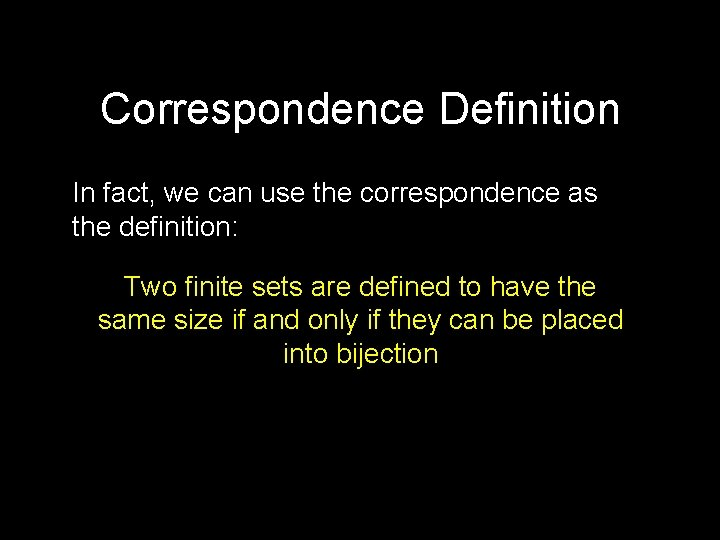Correspondence Definition In fact, we can use the correspondence as the definition: Two finite sets are defined to have the same size if and only if they can be placed into bijectionGeorg Cantor (1845 -1918)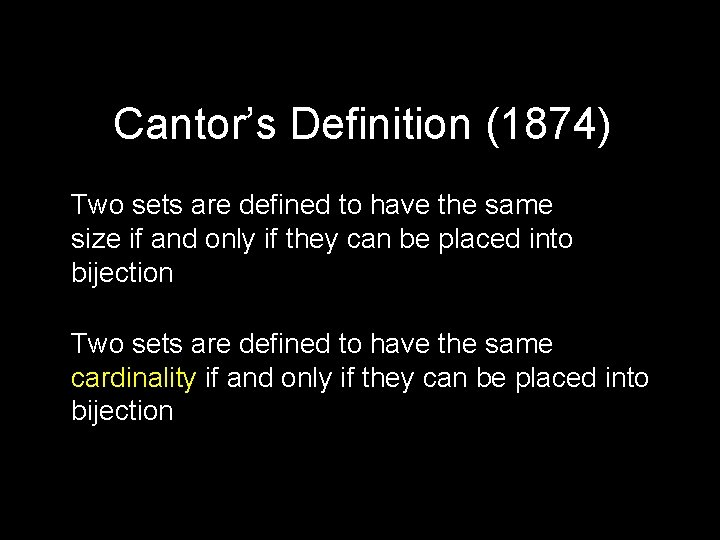Cantor’s Definition (1874) Two sets are defined to have the same size if and only if they can be placed into bijection Two sets are defined to have the same cardinality if and only if they can be placed into bijectionDo and E have the same cardinality? = { 0, 1, 2, 3, 4, 5, 6, 7, … } E = { 0, 2, 4, 6, 8, 10, 12, … } The even, natural numbers.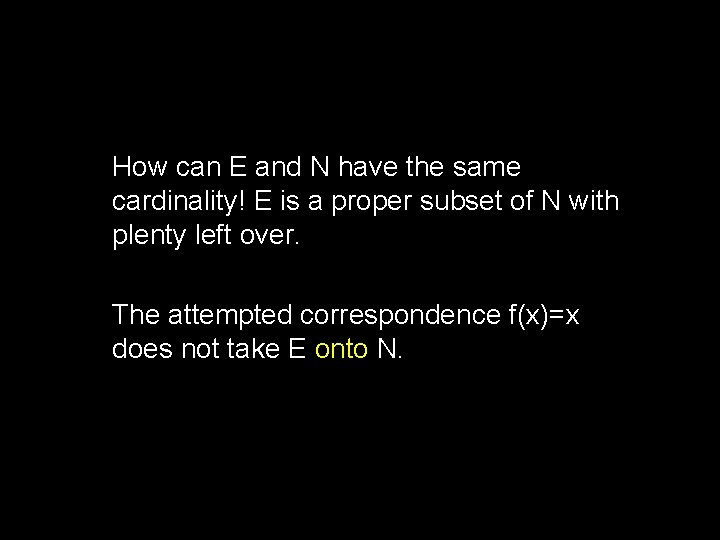How can E and N have the same cardinality! E is a proper subset of N with plenty left over. The attempted correspondence f(x)=x does not take E onto N.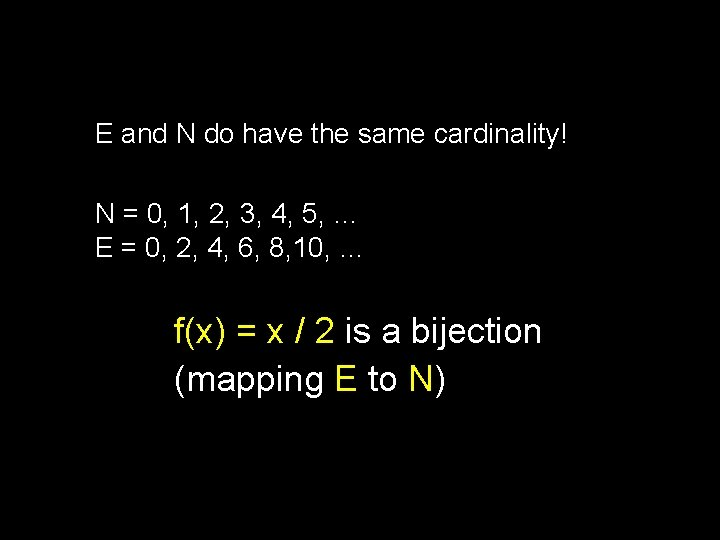E and N do have the same cardinality! N = 0, 1, 2, 3, 4, 5, … E = 0, 2, 4, 6, 8, 10, … f(x) = x / 2 is a bijection (mapping E to N)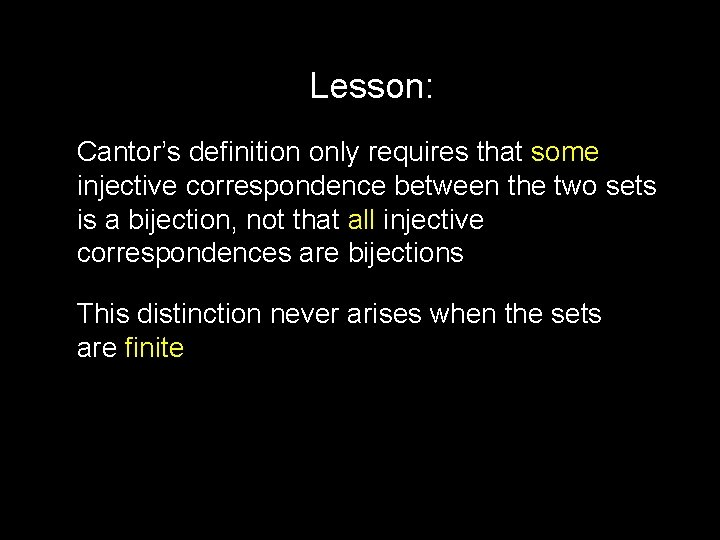Lesson: Cantor’s definition only requires that some injective correspondence between the two sets is a bijection, not that all injective correspondences are bijections This distinction never arises when the sets are finite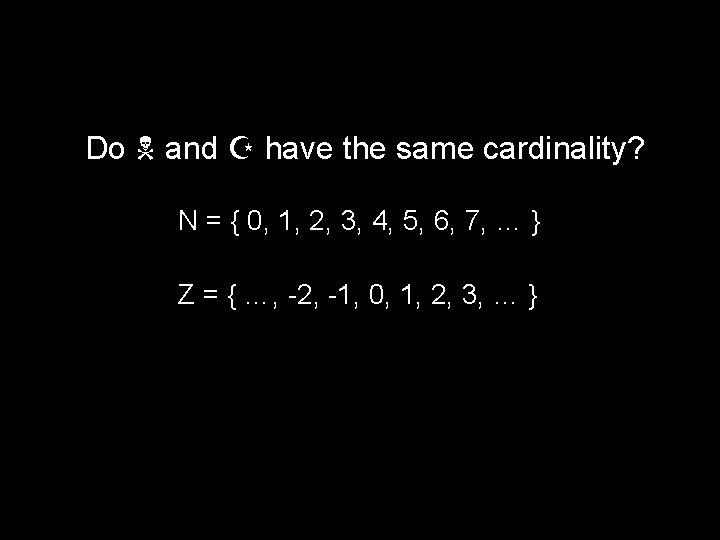Do and Z have the same cardinality? N = { 0, 1, 2, 3, 4, 5, 6, 7, … } Z = { …, -2, -1, 0, 1, 2, 3, … }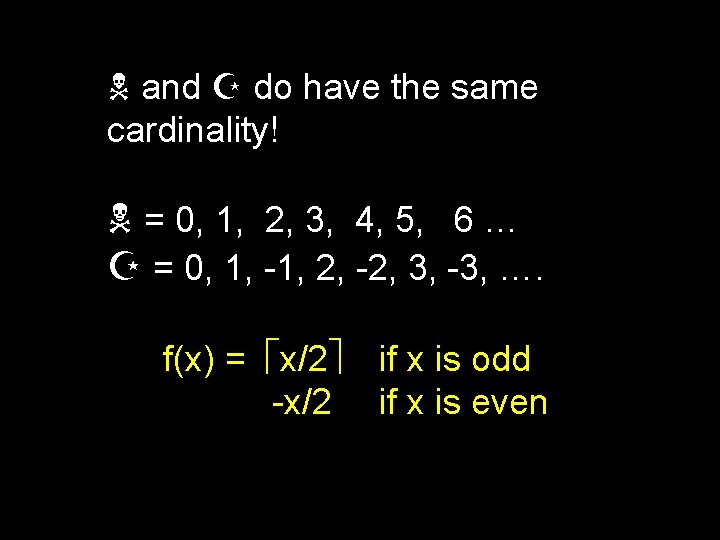and Z do have the same cardinality! = 0, 1, 2, 3, 4, 5, 6 … Z = 0, 1, -1, 2, -2, 3, -3, …. f(x) = x/2 -x/2 if x is odd if x is evenTransitivity Lemma: If f: A B is a bijection, and g: B C is a bijection. Then h(x) = g(f(x)) defines a function h: A C that is a bijection Hence, N, E, and Z all have the same cardinality.Do N and Q have the same cardinality? N = { 0, 1, 2, 3, 4, 5, 6, 7, …. } Q = The Rational NumbersHow could it be? ? The rationals are dense: between any two there is a third. You can’t list them one by one without leaving out an infinite number of themTheorem: N and N×N have the same cardinality … 4 3 The point (x, y) represents the ordered pair (x, y) 2 1 0 0 1 2 3 4 …Onto the Rationals!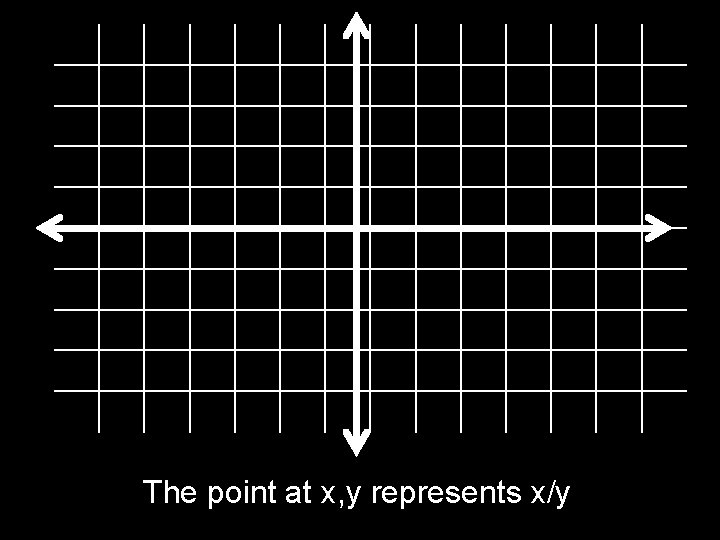The point at x, y represents x/y3 0 1 2 The point at x, y represents x/yCantor’s 1877 letter to Dedekind: “I see it, but I don't believe it! ”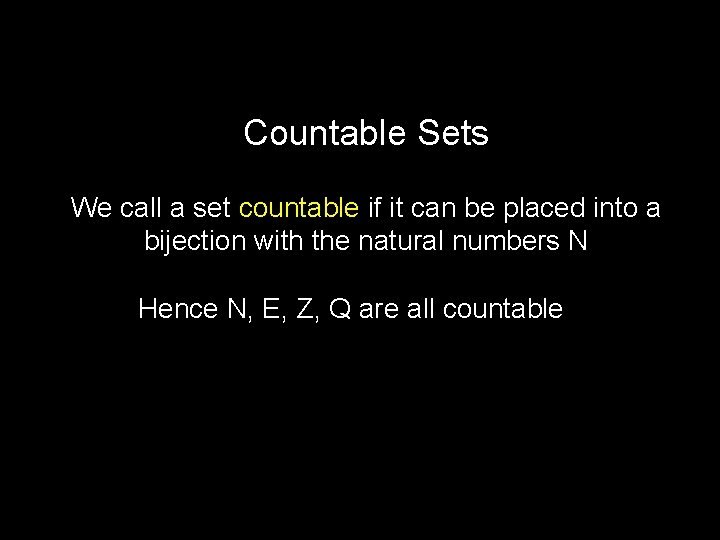Countable Sets We call a set countable if it can be placed into a bijection with the natural numbers N Hence N, E, Z, Q are all countable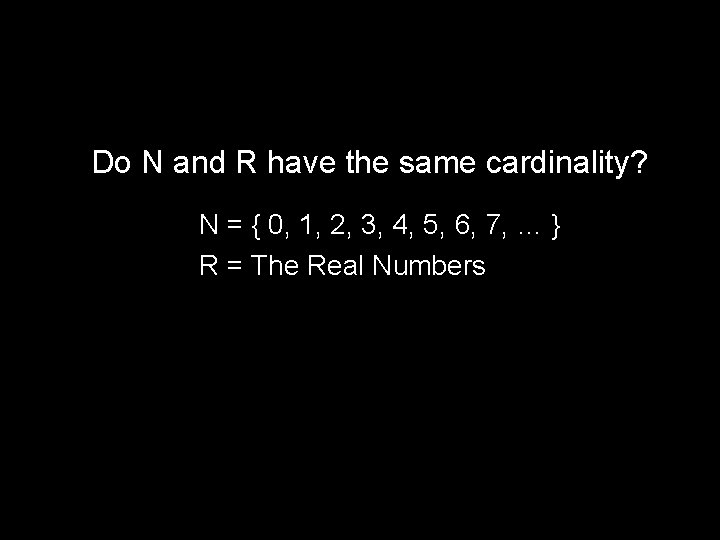Do N and R have the same cardinality? N = { 0, 1, 2, 3, 4, 5, 6, 7, … } R = The Real NumbersTheorem: The set R[0, 1] of reals between 0 and 1 is not countable Proof: (by contradiction) Suppose R[0, 1] is countable Let f be a bijection from N to R[0, 1] Make a list L as follows: 0: decimal expansion of f(0) 1: decimal expansion of f(1) … k: decimal expansion of f(k)Position after decimal point L Index 0 1 2 3 … 0 1 2 3 4 …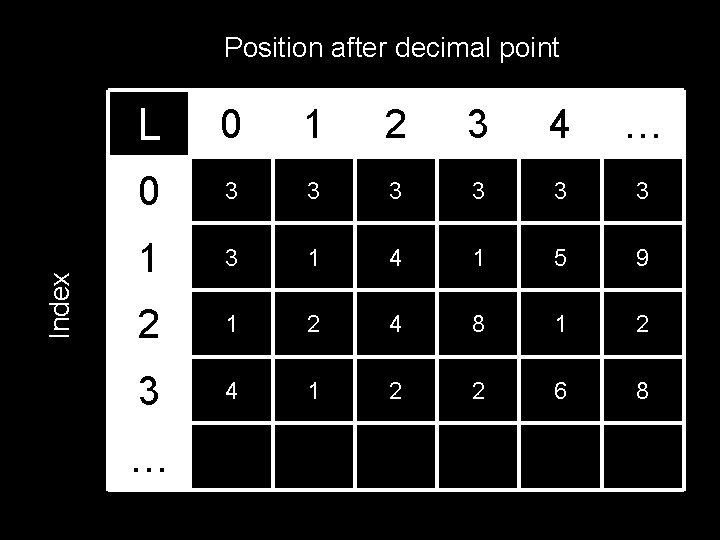Index Position after decimal point L 0 1 2 3 4 … 0 3 3 3 1 3 1 4 1 5 9 2 1 2 4 8 1 2 3 4 1 2 2 6 8 …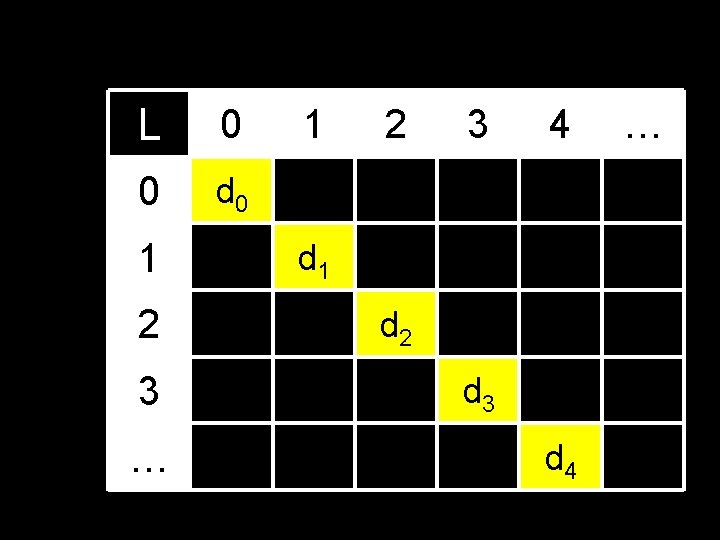L 0 0 d 0 1 2 3 … 1 2 3 4 d 1 d 2 d 3 d 4 …L 0 0 d 0 1 1 2 3 4 d 1 d 2 2 d 3 3 … … Define the following real number Confuse. L = 0. C 0 C 1 C 2 C 3 C 4 C 5 … Ck= 5, if dk=6 6, otherwiseDiagonalized! By design, Confuse. L can’t be on the list L! Confuse. L differs from the kth element on the list L in the kth position. This contradicts the assumption that the list L is complete; i. e. , that the map f: N to R[0, 1] is onto.The set of reals is uncountable! (Even the reals between 0 and 1)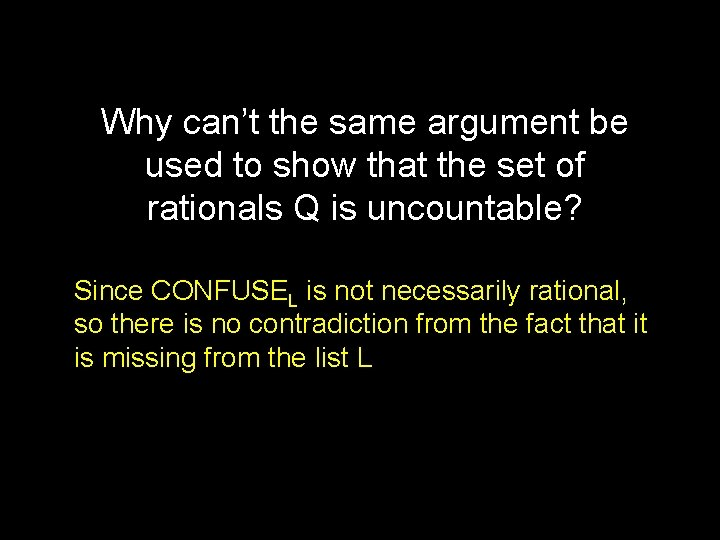Why can’t the same argument be used to show that the set of rationals Q is uncountable? Since CONFUSEL is not necessarily rational, so there is no contradiction from the fact that it is missing from the list LBack to the questions we were asking earlierAre all reals describable? Are all reals computable? We saw that computable describable, but do we also have describable computable?Standard Notation S = Any finite alphabet Example: {a, b, c, d, e, …, z} S* = All finite strings of symbols from S including the empty string e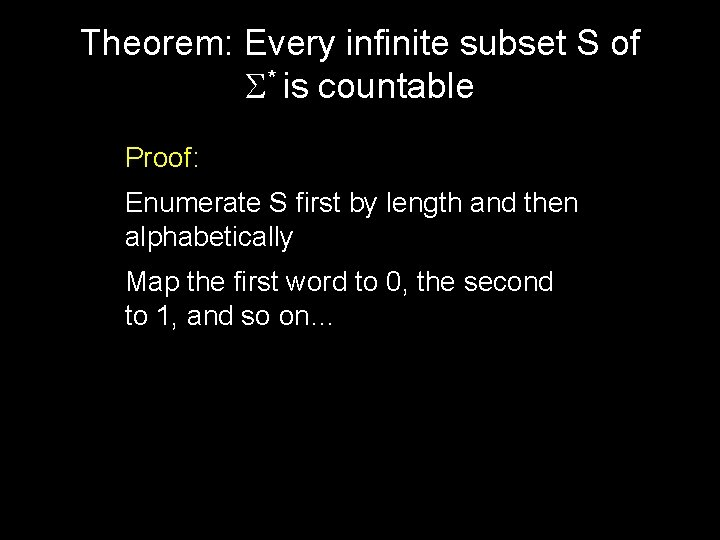Theorem: Every infinite subset S of S* is countable Proof: Enumerate S first by length and then alphabetically Map the first word to 0, the second to 1, and so on…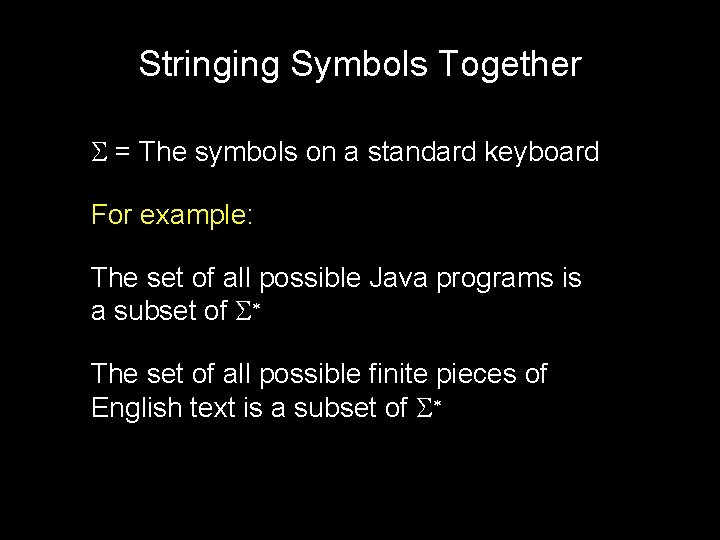Stringing Symbols Together S = The symbols on a standard keyboard For example: The set of all possible Java programs is a subset of S* The set of all possible finite pieces of English text is a subset of S*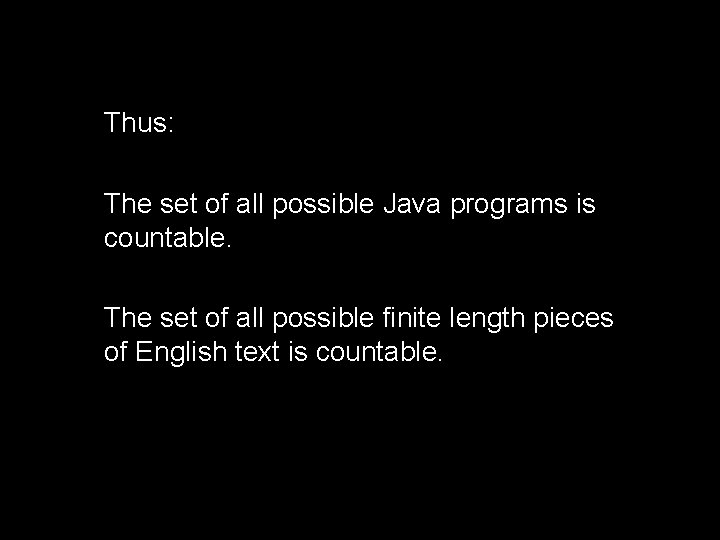Thus: The set of all possible Java programs is countable. The set of all possible finite length pieces of English text is countable.There are countably many Java programs and uncountably many reals. Hence, most reals are not computable!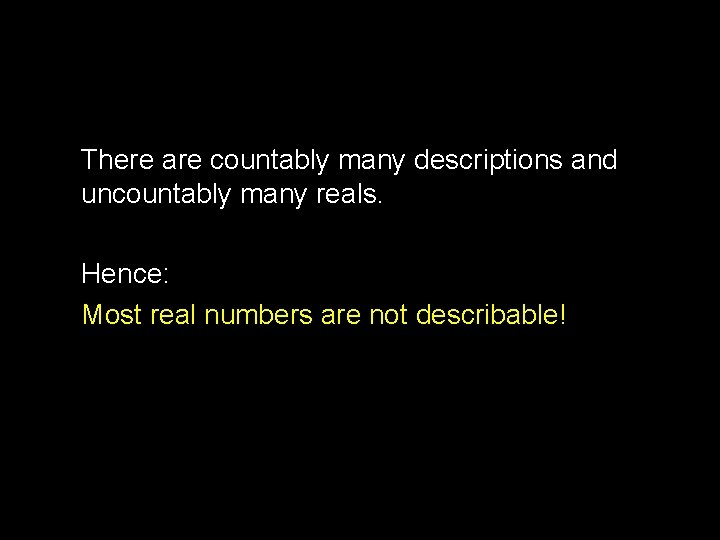There are countably many descriptions and uncountably many reals. Hence: Most real numbers are not describable!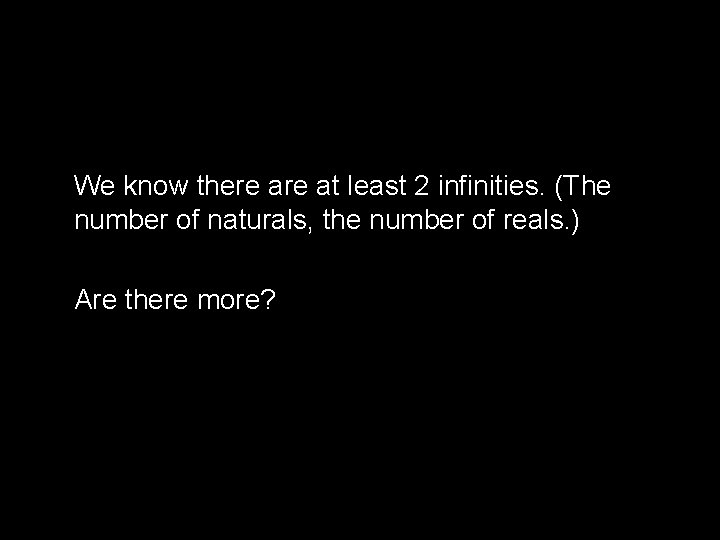We know there at least 2 infinities. (The number of naturals, the number of reals. ) Are there more?Definition: Power Set The power set of S is the set of all subsets of S. The power set is denoted as P(S) Proposition: If S is finite, the power set of S has cardinality 2|S|Theorem: S can’t be put into bijection with P(S) S A B { C } C { A } { B } {A, C} {B, C} {A, B, C } Suppose f: S->P(S) is a bijection. Let CONFUSEf = { x | x S, x f(x) } Since f is onto, there exists a y S such that f(y) = CONFUSEf. Is y in CONFUSEf? YES: Definition of CONFUSEf implies no NO: Definition of CONFUSEf implies yes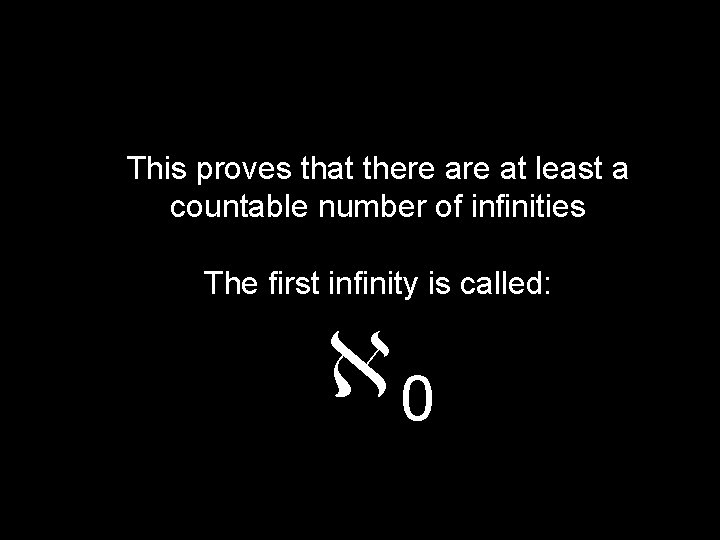This proves that there at least a countable number of infinities The first infinity is called: 0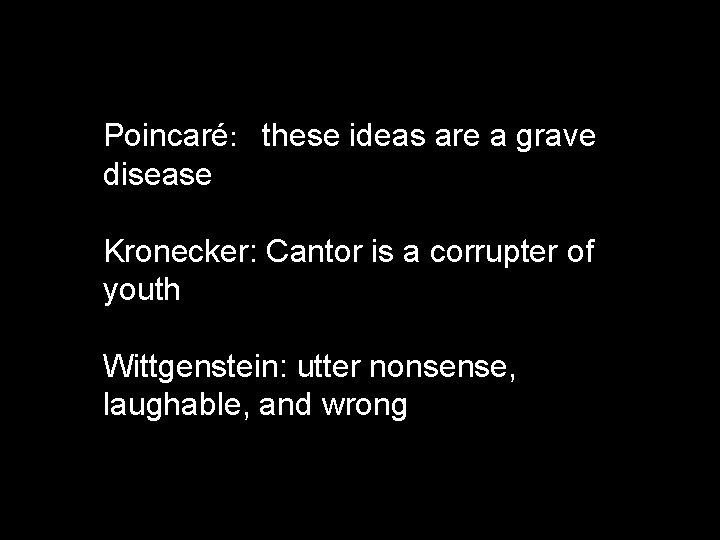Poincaré: these ideas are a grave disease Kronecker: Cantor is a corrupter of youth Wittgenstein: utter nonsense, laughable, and wrong0, 1, 2, … Cantor wanted to show that the number of reals was 1Cantor called his conjecture that 1 was the number of reals the “Continuum Hypothesis. ” However, he was unable to prove it. This helped fuel his depression.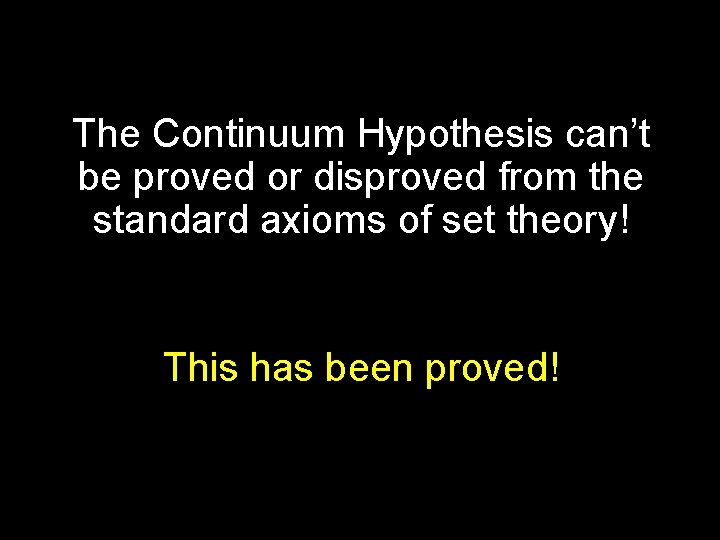The Continuum Hypothesis can’t be proved or disproved from the standard axioms of set theory! This has been proved!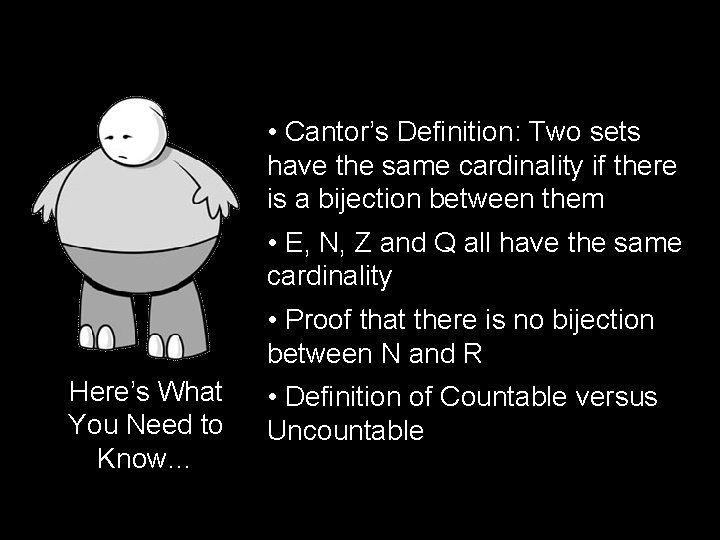• Cantor’s Definition: Two sets have the same cardinality if there is a bijection between them • E, N, Z and Q all have the same cardinality • Proof that there is no bijection between N and R Here’s What You Need to Know… • Definition of Countable versus Uncountable